Now, sometimes questions will give you a set of inequalities, rather than a set of equations. For example,In these problems, we are not looking for specific solutions forand. This is because there are many solutions to the above equations. For example,is a solution and so isRather, we want to characterize, in a general way, what the complete set of solutions looks like.

The easiest way to do this is by graphing the above equations. If we graph them, we get: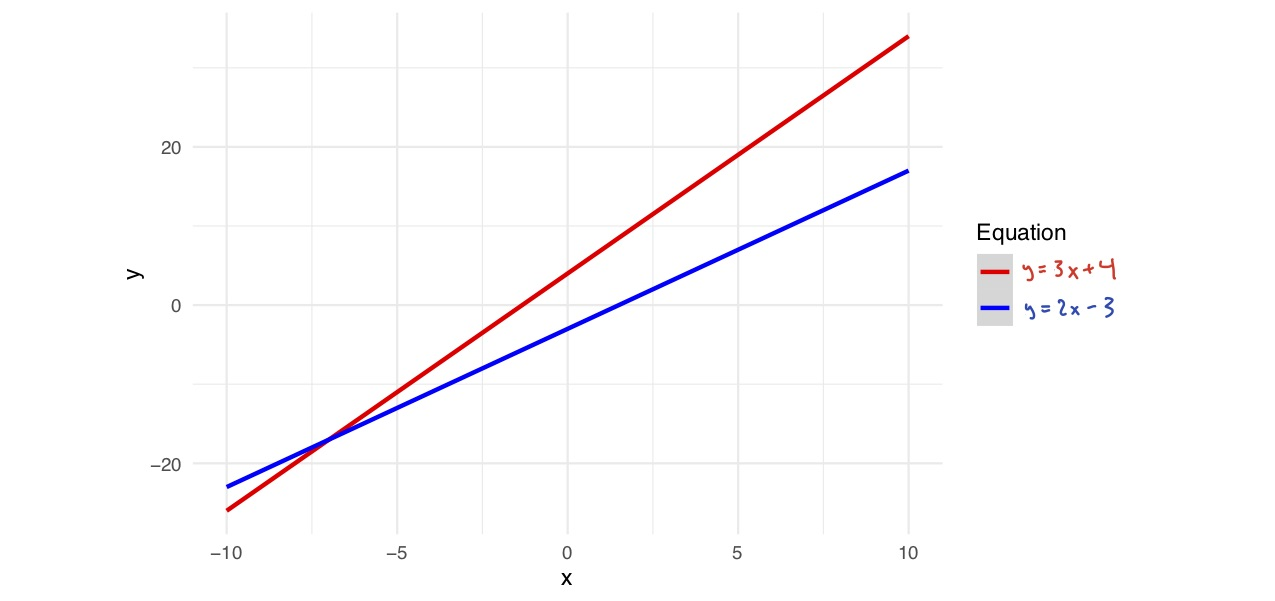Now, we want to determine where the inequalities hold. So suppose we’re interested in the first inequality,Where will this be true?

It will be true below (and on) the lineWe can mark this on our diagram by shading in that region: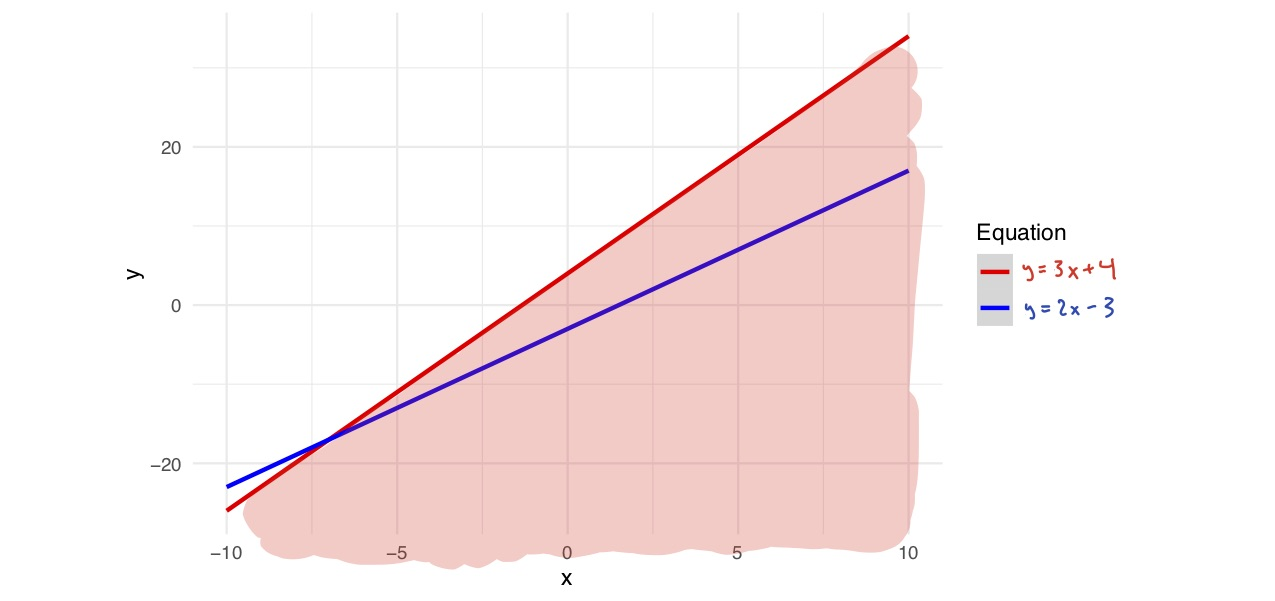And typically, we use a solid line to designate.

Now, what about the second inequality,? Typically, we use a dotted line to designate. So, we get: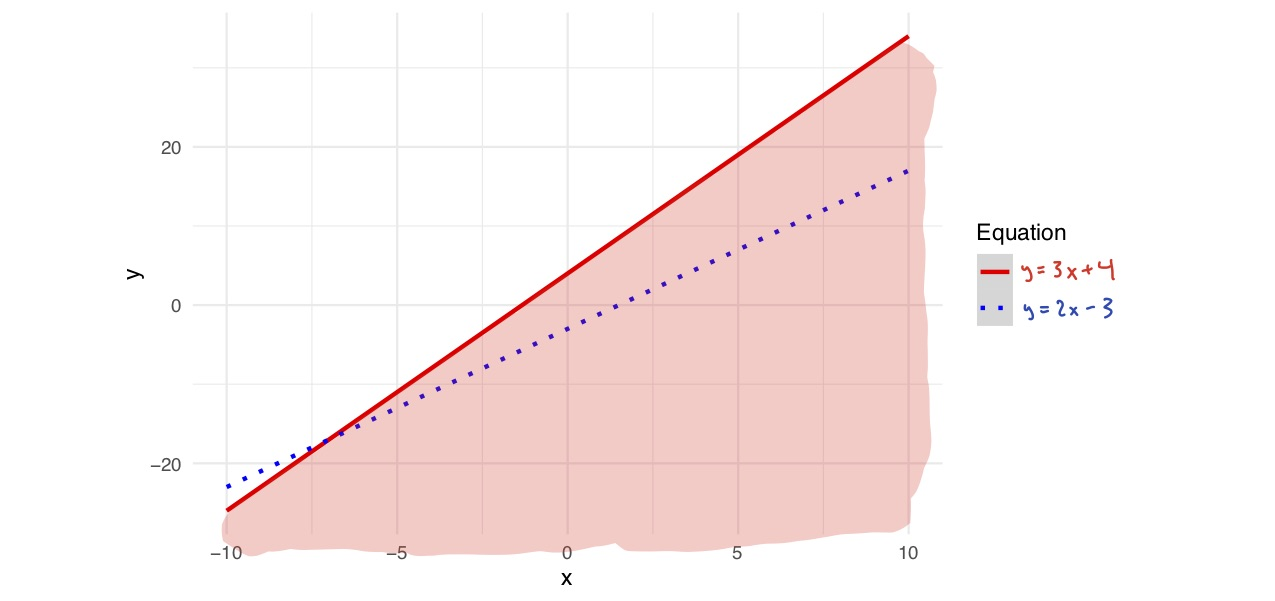And now we can go to our graph and we ask: where isgreater than? We have this line showing wheresomust be greater thanwhenever we are above this line. (Alternatively, if you’re unsure which side of the line satisfies the inequality, pick a point on each side and test whether it satisfies the inequality).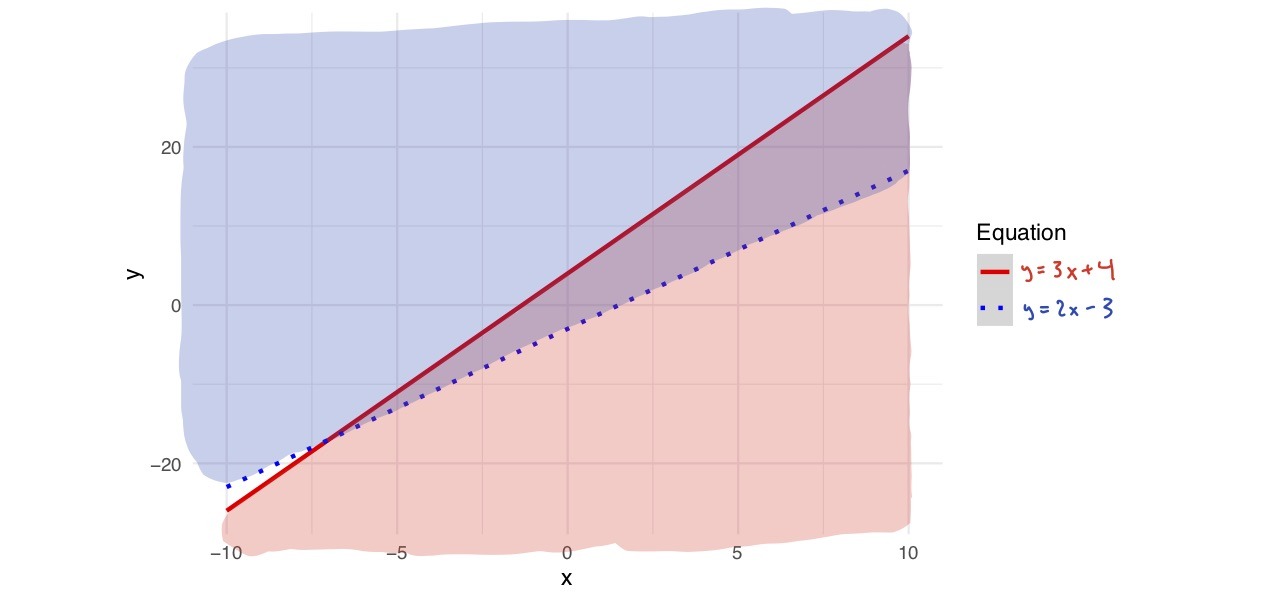Now, where will both of these inequalities be true? Well recall that the red shading indicates the first inequality is true and the blue shading indicates the second inequality is true. So the inequalities are both true at any point that is shaded both red and blue. So the solutions to these inequalities are just the points in this region that is shaded both blue and red. In other words: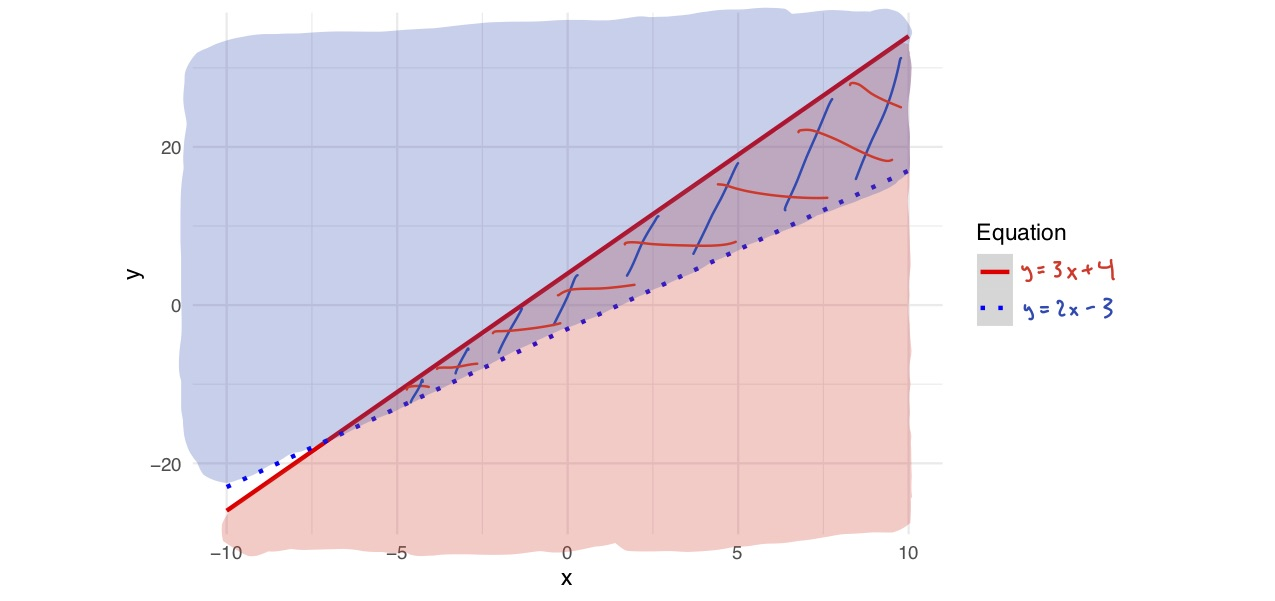Now sometimes your equations may not seem easy to graph. For example, you might getBut with some algebra, we can always convert it into slope-intercept form:and then we can graph it more easily.

Finally, sometimes your inequalities will have no solution. For example:has no solution, as can be verified by looking at the graph: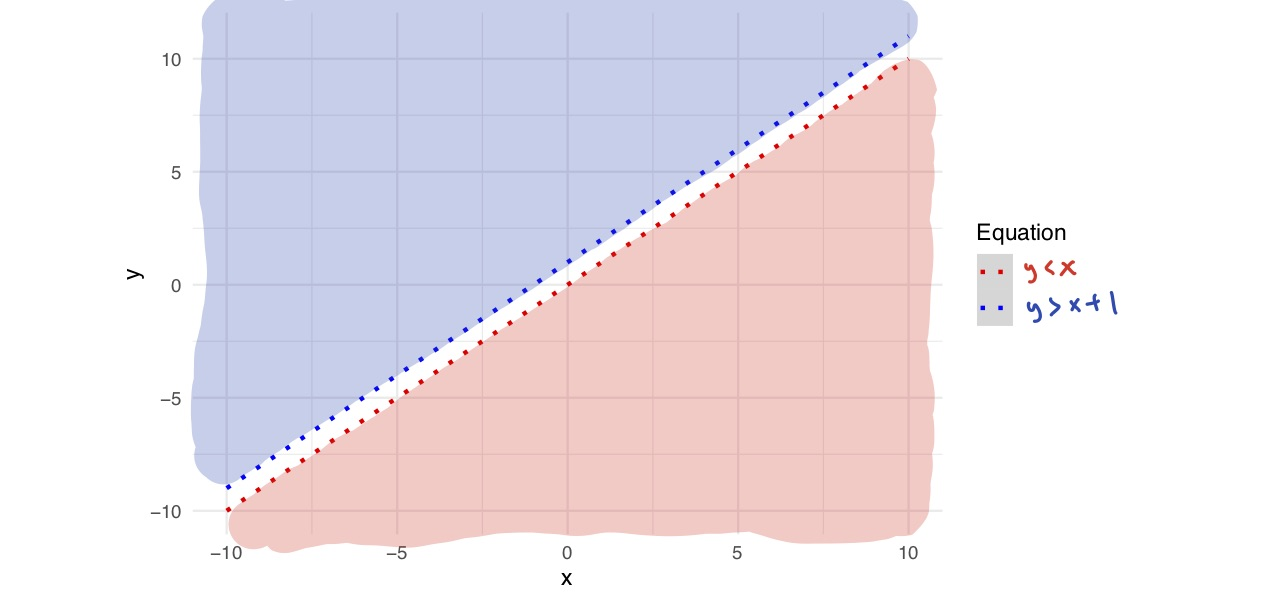and noticing that there is no point that falls into both shaded regions. In this case, we just say that the system has no solution.

Finally, it is important to know how to handle equations likeTo address these cases, we need to know how to graph a function likeTo graph that function, we just draw a vertical line at any point with an x-coordinate of 3, as seen here: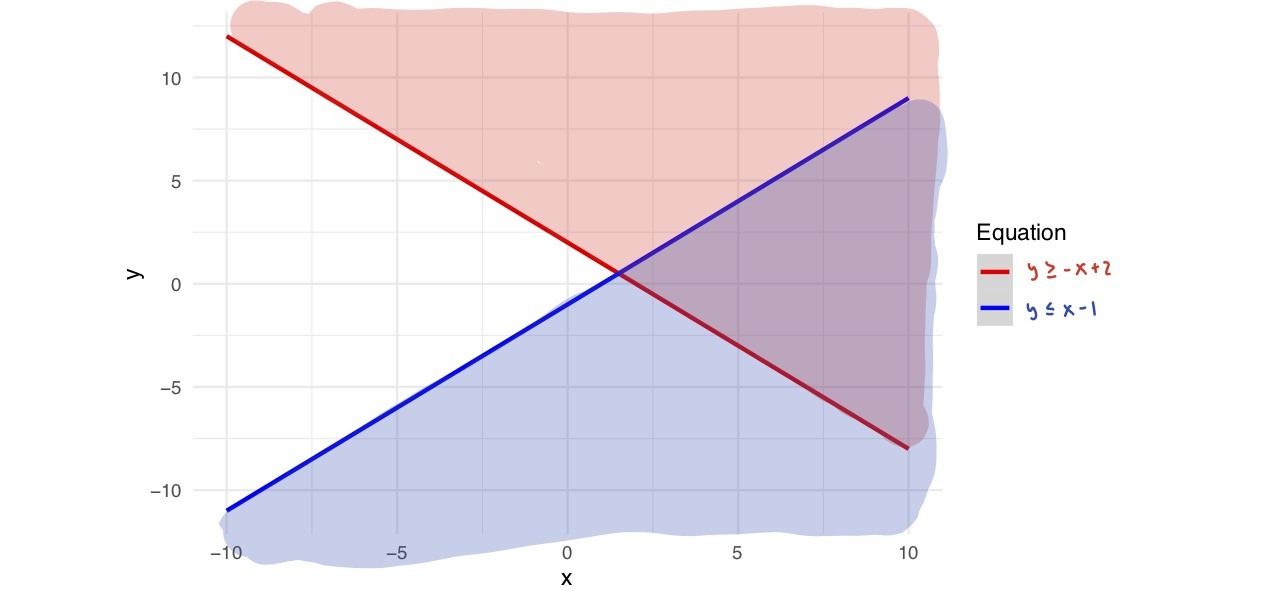And to tell which side of the line to shade in, we can just try a point on each side.satisfieswhereasdoes not. Thus, we shade in the side to the right, and we use a solid line because this is a weak inequality (i.e. includes the possibility).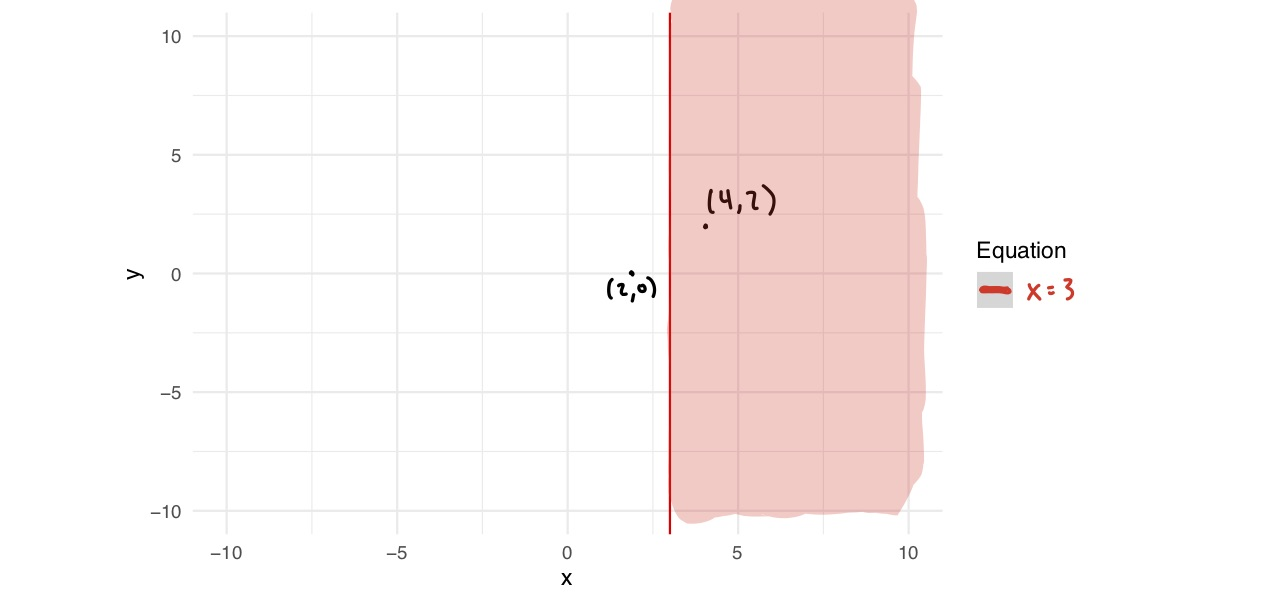Practice Problems

Graph the solutions to the following:

1.2.3.